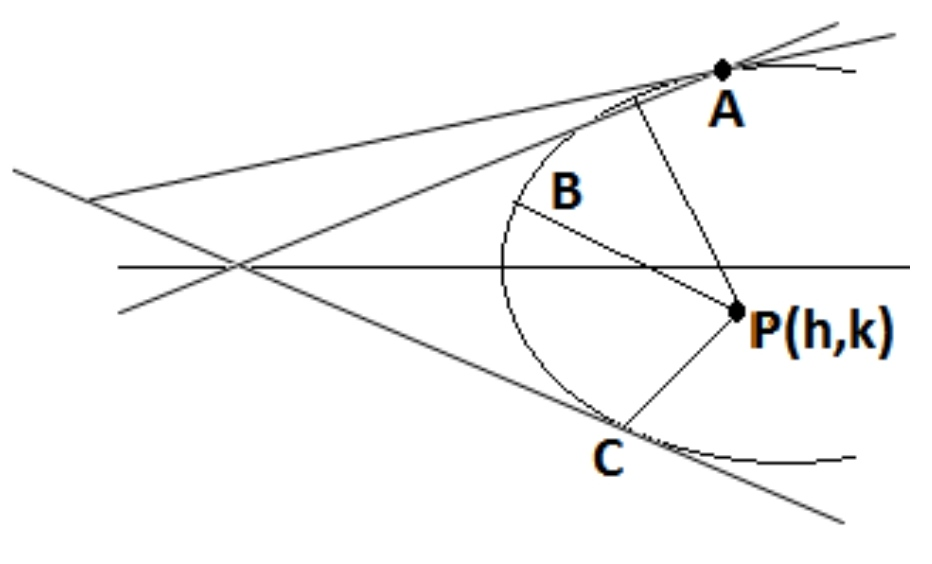# Co-Normal Parabolic CentroidCentroid of triangle formed by three Co-Normal points $A(x_1, y_1), B(x_2, y_2) \text{ and } C(x_3, y_3)$ on parabola $y^2 = 8x$ is $G(4, 0)$. Two of the three points $A, B \text{ and } C$ lie above the $\text{x-axis}$ $\underline{\text{or}}$ one of the point lies on x-axis. The normals drawn on parabola $y^2 = 8x$ at $A, B \text{ and } C$ are concurrent.

If $h = a$ and $k \gt b$, enter answer as $a^2 + b^2$.

All of my problems are original

×